Close

# OpenFOAM 2.2.0: Numerical Methods

When solving transport equations, e.g. for enthalpy h, the material time derivative is expressed in terms of a spatial time derivative and convection, i.e.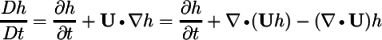For numerical solution of incompressible flows,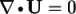at convergence, at which point the third term on the right hand side is zero. Before convergence is reached, however,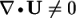and in some circumstances it is better to include the term within a numerical solution because it helps maintain boundedness of the solution variable and promotes better convergence.

In particular, for steady-state it is necessary to use the bounded form, equivalent to

```fvm::div(phi, h) - fvm::Sp(fvc::div(phi), h)
```

For transient solutions, it is usually better to implement only the term

`fvm::div(phi, h)`

Where transport equations are buried within models, e.g. turbulence, it is better if the users can select the form of the discretisation to suit the problem they are solving, i.e. steady or transient, than having one form hard-coded.

Prior to this version of OpenFOAM, the form of convective derivative was hard-coded into transport equations. In version 2.2.0, we have introduced a bounded form of discretisation which, when applied to a convective derivative such as `fvm::div(phi, h)`, will include a component for the `-fvm::Sp(fvc::div(phi), h)` term. Users can verify, by referring to steady-state example cases in OpenFOAM, that the bounded form of discretisation is adopted as expected; the `divSchemes` sub-dictionary in fvSchemes for the steady-state motorBike tutorial looks like:

```divSchemes
{
default         none;
div(phi,k)      bounded Gauss upwind;
div(phi,omega)  bounded Gauss upwind;
}
```

Compressible solvers for transient problems generally use the PIMPLE algorithm, which supports partial convergence of intermediate iterations. The solution may benefit from the use of the bounded form of convection but, in such cases, the corresponding bounded time derivative must also be included, since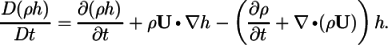In other words, the `- fvm::Sp(fvc:ddt(rho), h)` must be included through a `bounded` version of `ddtSchemes`, e.g.:

```ddtSchemes
{
default         Euler;
ddt(rho,h)     bounded Euler;
}

divSchemes
{
...
}
```

#### Example

• motorbike
`\$FOAM``_TUTORIALS/incompressible/simpleFoam/motorBike`

## Cell Value Reconstruction

This release includes a new “face-volume” weighting for reconstruction of cell values from fluxes using the `fvc::reconstruct` function. The new weighting improves robustness of solvers on poor-quality meshes. This is particularly important for VoF and other multiphase solvers in which the momentum sources are all reconstructed to maintain force balances.

## Implicit Region Coupling

For multi-region cases, such as when modelling conjugate heat transfer between multiple fluids and solids, the solution of the energy system when using a segregated approach may require many iterations to converge. This is partly due to the explicit treatment of the thermal boundaries coupling the regions.

A new framework for the implicit solution of coupled thermal boundaries has been implemented, allowing a closer coupling between the solid and fluid regions. From a user perspective, the only change necessary is to employ a new type of boundary condition. Currently, this is still a work-in-progress, and under active development.

#### Source code

• regionCoupled directories
`\$FOAM``_SRC/regionCoupled`
`\$FOAM``_SRC/meshTools/regionCoupled`

## Schemes

A new numerical scheme, called `CoBlended`, has been implemented that blends two different schemes based on the local flow Courant number. The functional form of the blending factor/weight, `w` is given by: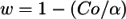where `Co` is the local Courant number, and `alpha` is a scheme coefficient. Accordingly, for large alpha there is a bias towards the first scheme, and for small alpha, the bias is towards the second scheme.

6th March 2013